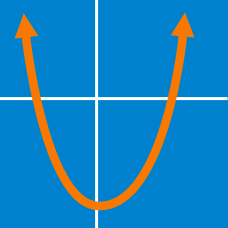Algebra

# Solve by Quadratic Formula

What is the root of the quadratic equation

$x^2 + 6 x +9 = 0?$

What are the roots of the quadratic equation

$x^2 -2 x -48 = 0?$

What are the roots of the quadratic equation

$x^2 - 2 x -5 = 0?$

From the top of a building of 960 feet, Tommy threw a coin upwards. The height of the coin from the ground $t$ seconds after it has been thrown is given by

$h = - 16t^2 + 8 t + 960.$

How long (in seconds) would it take the coin to hit the ground?

What are the roots of the quadratic equation

$0.5x^2 + 0.6 x -0.2 = 0?$

×